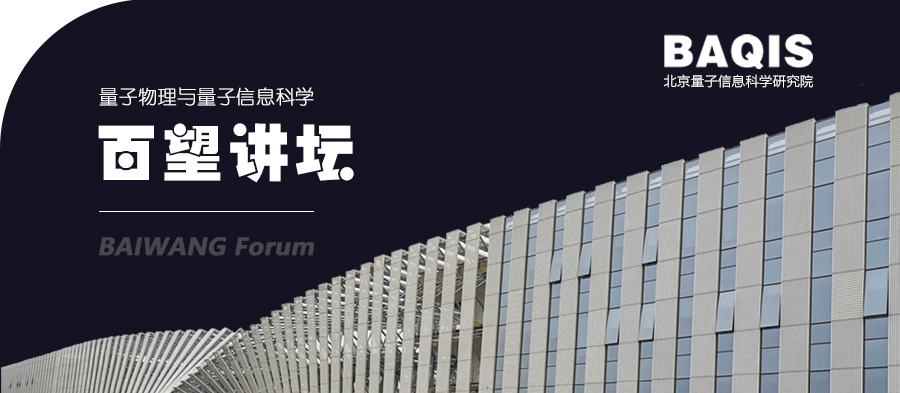####### Baiwang Forum 28: Quantum-classical entangled approach with tensor networks to investigating quantum spin liquid

2022/07/07Date and Time:  7-July-2022  14:00

Speaker:  Prof. Tsuyoshi Okubo, project associate professor at Graduate School of Science, University of Tokyo

Host: Takahiro Misawa ( BAQIS）

Title: Quantum-classical entangled approach with tensor networks to investigating quantum spin liquid

Abstract:

To represent a quantum many-body state, we need to treat huge vectors in exponentially increasing dimensions as we increase the number of particles. Such an exponentially large vector space is a fundamental difficulty in treating quantum many-body problems in classical computers. However, when we use a well-controlled quantum system, such as a quantum computer, we may solve quantum many-body problems with a cost polynomial of the number of particles. In recent years, to use a noisy quantum computer for quantum many-body problems, the variational quantum eigensolver (VQE)  has attracted much interest. In the VQE approach, a quantum many-body state is represented as a quantum circuit, and we optimize circuit parameters to minimize the energy expectation value. However, it is still unclear whether the VQE approach can exceed classical computation or not for practical quantum many-body problems.

In this talk, We will discuss the possibility of using tensor network representations of quantum many-body states to design an efficient quantum circuit suitable for near future noisy quantum computers. As a concrete example, we will consider the spin liquid state in the honeycomb lattice Kitaev model . We show that a simple tensor network state can capture quantitative properties of the spin liquid, and by adding short-range excitations, we can systematically improve its energy expectation value . A similar procedure can be applied to the VQE approach by representing tensor network states as quantum circuits. We will discuss that through this approach, we can efficiently optimize the infinite system by solving an optimization problem in small clusters.

 A. Peruzzo, J. McClean, P. Shadbolt, et al., Nat. Commun. 5, 4213 (2014).

 A. Kitaev, Ann. Phys. 321, 2 (2006).

 H.-Y. Lee, R. Kaneko, T. Okubo, and N. Kawashima, Phys. Rev. Lett. 123, 087203 (2019).